## Some 3D renderings of the Mandelbrot set.

As mentioned previously, using geometric algebra we can convert the iterative equation for the Mandelbrot set from complex number form
\begin{equation}
z \rightarrow z^2 + c,
\end{equation}
to an equivalent vector form
\begin{equation}
\mathbf{x} \rightarrow \mathbf{x} \mathbf{e} \mathbf{x} + \mathbf{c},
\end{equation}
where $$\mathbf{e}$$ represents the x-axis (say). Geometrically, each iteration takes $$\mathbf{e}$$ and reflects it about the direction of $$\mathbf{x}$$, then scales that by $$\mathbf{x}^2$$ and adds $$\mathbf{c}$$.

To get the usual 2D Mandelbrot set, one iterates with vectors that lie only in the x-y plane, but we can treat the Mandelbrot set as a 3D solid if we remove the x-y plane restriction.

Last time I animated slices of the 3D set, but after a whole lot of messing around I managed to save the data for all the interior points of the 3D set in netCDF format, and render the solid using Paraview. Paraview has tons of filters available, and experimenting with them is pretty time consuming, but here are some initial screenshots of the 3D Mandelbrot set: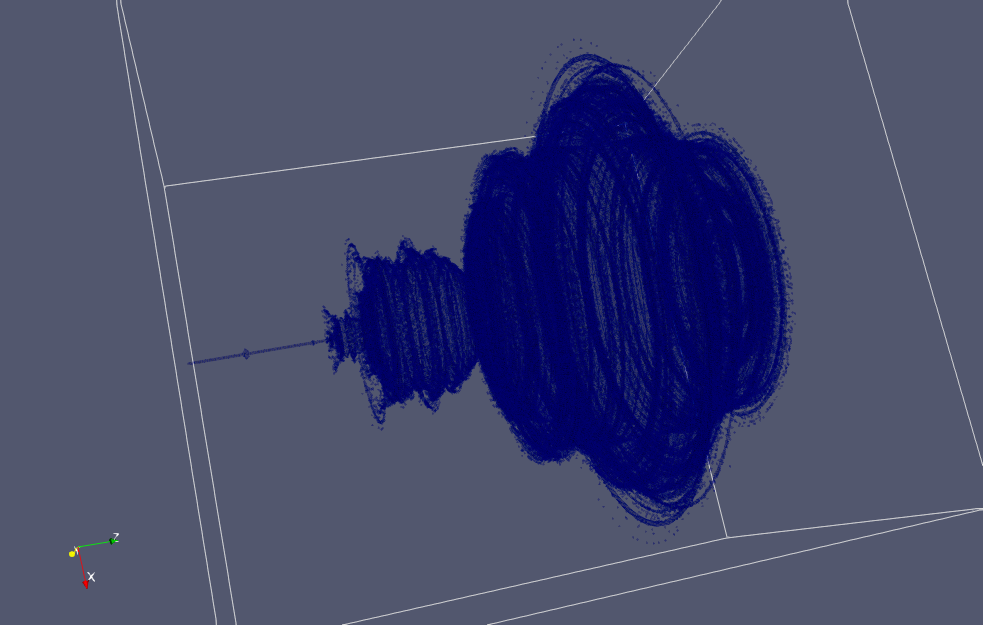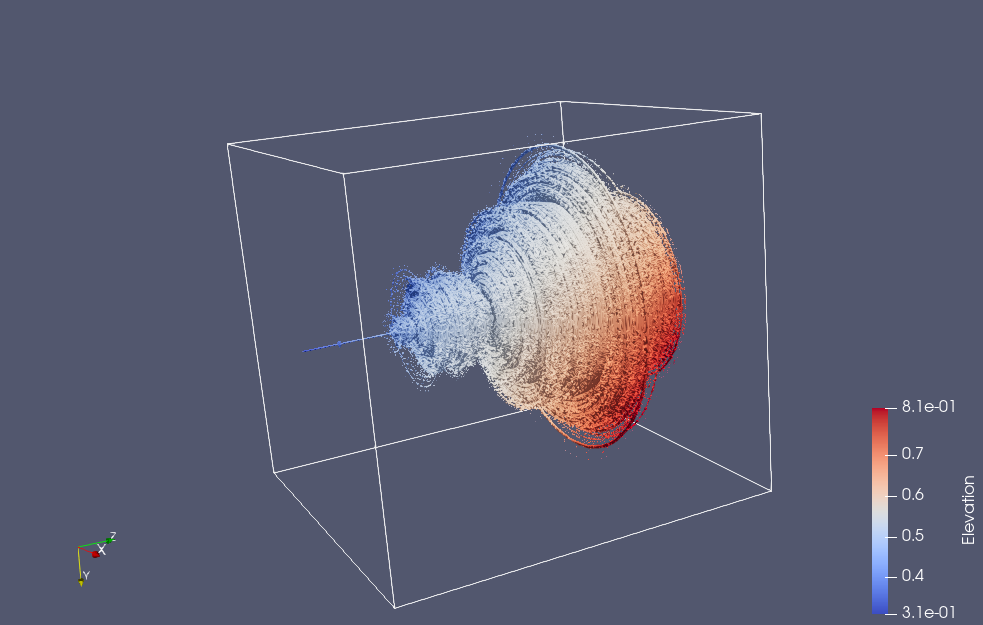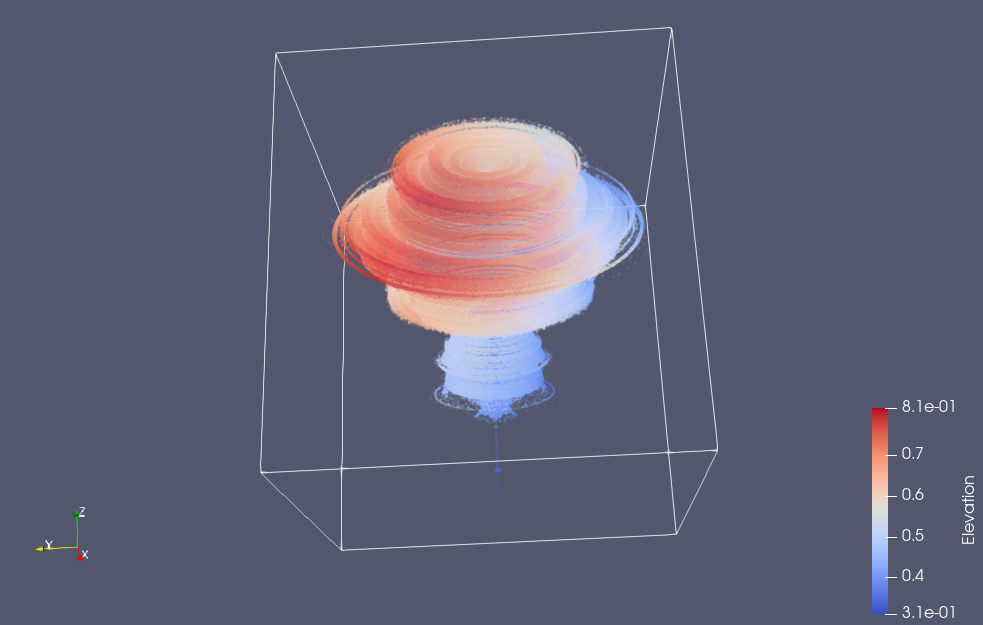It’s interesting that much of the characteristic detail of the Mandelbrot set is not visible in the 3D volume, but if we slice that volume, you can then you can see it again.  Here’s a slice taken close to the z=0 plane (but far enough that the “CN tower” portion of the set is not visible)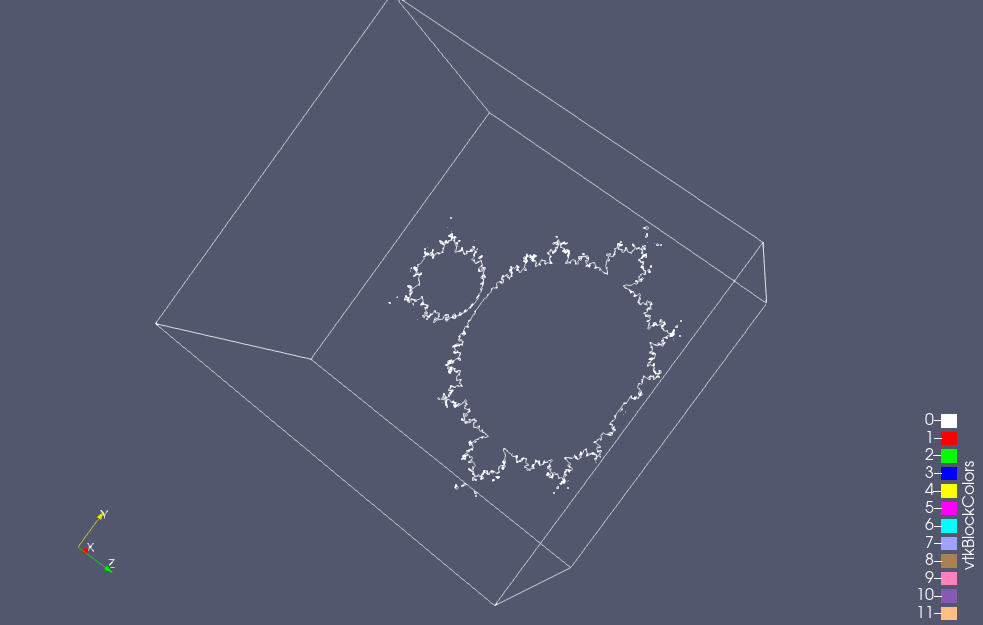You can also see some of that detail if the opacity of the rendering is turned way down: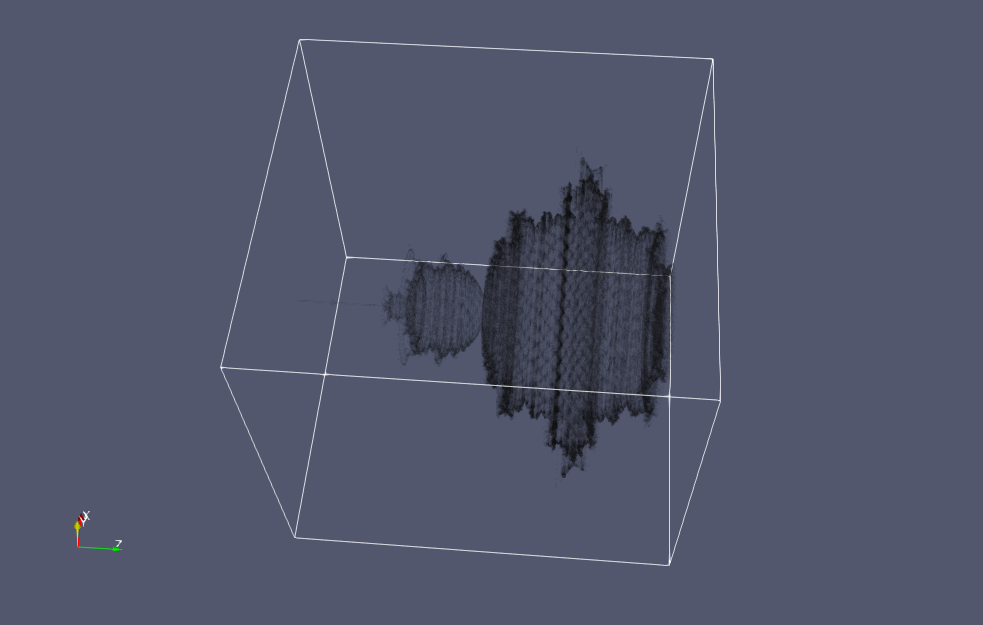If you look carefully at the images above, you’ll see that the axis labels are wrong.  I think that I’ve screwed up one of the stride related parameters to my putVar call, and I end up with x+z transposed in the axes labels when visualized.

## What’s in the pipe.

It’s been a while since I did any math or physics writing. This is the first post in a series where I plan to work my way systematically from an introduction of vectors, to the axioms of geometric algebra.  I plan to start with an introduction of vectors as directed “arrows”, building on that to discuss coordinates, tuples, and column matrix representations, and representation independent ideas. With those basics established, I’ll remind the reader about how generalized vector and dot product spaces are defined and give some examples. Finally, with the foundation of vectors and vector spaces in place, I’ll introduce the concept of a multivector space, and the geometric product, and start unpacking the implications of the axioms that follow naturally from this train of thought.

The applications that I plan to include in this series will be restricted to Euclidean spaces (i.e. where length is given by the Pythagorean law), primarily those of 2 and 3 dimensions.  However, it will be good to also lay the foundations for the non-Euclidean spaces that we encounter in relativistic electromagnetism (there is actually no other kind), and in computer graphics applications of geometric algebra, especially since we can do so nearly for free.  I plan to try to introduce the requisite ideas (i.e. the metric, which allows for a generalized dot product) by discussing Euclidean non-orthonormal bases.  Such bases have applications in condensed matter physics where there are useful for modelling crystal and lattice structure, and provide a hands conceptual bridge to a set of ideas that might otherwise seem abstract and without “real world” application.

## Motivation.

Many introductions to geometric algebra start by first introducing the dot product, then bivectors and the wedge product, and eventually define the product of two vectors as the synthetic sum of the dot and wedge
\begin{equation}\label{eqn:multivector:20}
\Bx \By = \Bx \cdot \By + \Bx \wedge \By.
\end{equation}
It takes a fair amount of work to do this well. In the seminal work  a few pages are taken for each of the dot and wedge products, showing the similarities and building up ideas, before introducing the geometric product in this fashion. In  the authors take a phenomenal five chapters to build up the context required to introduce the geometric product.  I am not disparaging the authors for taking that long to build up the ideas, as their introduction of the subject is exceedingly clear and thorough, and they do a lot more than the minumum required to define the geometric product.

The strategy to introduce the geometric product as a sum of dot and wedge can result in considerable confusion, especially since the wedge product is often defined in terms of the geometric product
\begin{equation}\label{eqn:multivector:40}
\Bx \wedge \By =
\inv{2} \lr{
\Bx \By – \By \Bx
}.
\end{equation}
The whole subject can appear like a chicken and egg problem. I personally found the subject very confusing initially, and had considerable difficulty understanding which of the many identities of geometric algebra were the most fundamental. For this reason, I found the axiomatic approach of  very refreshing. The cavaet with that work is that is is exceptionally terse, as they jammed a reformulation of most of physics using geometric algebra into that single book, and it would have been thousands of pages had they tried to make it readable by mere mortals.

When I wrote my own book on geometric algebra, I had the intuition that the way to introduce the subject ought to be like the vector space in abstract linear algebra. The construct of a vector space is a curious and indirect way to define a vector. Vectors are not defined as entities, but simply as members of a vector space, a space that is required to have a set of properties. I thought that the same approach would probably work with multivectors, which could be defined as members of a multivector space, a mathematical construction with a set of properties.

I did try this approach, but was not fully satisfied with what I wrote. I think that dissatisfaction was because I tried to define the multivector first. To define the multivector, I first introduced a whole set of prerequisite ideas (bivector, trivector, blade, k-vector, vector product, …), but that was also problematic, since the vector multiplication idea required for those concepts wasn’t fully defined until the multivector space itself was defined.

My approach shows some mathematical cowardness. Had I taken the approach of the vector space fully to heart, the multivector could have been defined as a member of a multivector space, and all the other ideas follow from that. In this multi-part series, I’m going to play with this approach anew, and see how it works out.  If it does work, I’ll see if I can incorporate this approach into a new version of my book.

## Review and background.

In this series, I’m going to assume a reader interested in geometric algebra, is probably also familiar with a wide variety of concepts, including but not limited to

• vectors,
• coordinates,
• matrices,
• basis,
• change of basis,
• dot product,
• real and complex numbers,
• rotations and translations,
• vector spaces, and
• linear transformations.

Despite those assumptions, as mentioned above, I’m going to attempt to build up the basics of vector representation and vector spaces in a systematic fashion, starting from a very elementary level.

My reasons for doing so are mainly to explore the logical sequencing of the ideas required.  I’ve always found well crafted pedagogical sequences rewarding, and will hopefully construct one here that is appreciated by anybody who chooses to follow along.

## Next time.

As preparation for the next article in this series, the reader is asked to watch a short lesson from Vector, not so supervillain extraordinaire (Despicable Me).

## References

 C. Doran and A.N. Lasenby. Geometric algebra for physicists. Cambridge University Press New York, Cambridge, UK, 1st edition, 2003.

 L. Dorst, D. Fontijne, and S. Mann. Geometric Algebra for Computer Science. Morgan Kaufmann, San Francisco, 2007.

 D. Hestenes. New Foundations for Classical Mechanics. Kluwer Academic Publishers, 1999.